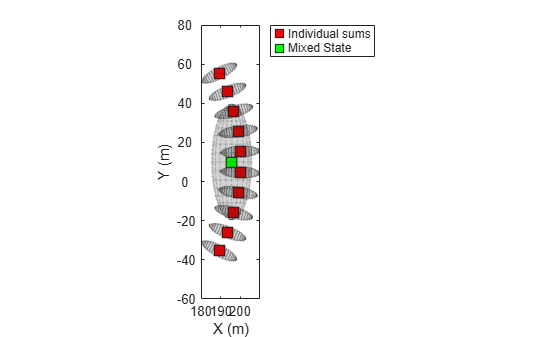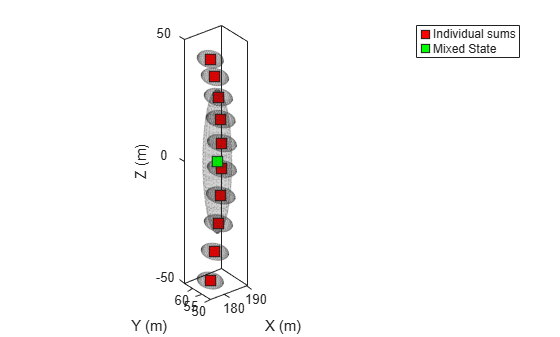# initapekf

Constant velocity angle-parameterized EKF initialization

## Syntax

``filter = initapekf(detection)``
``filter = initapekf(detection,numFilters)``
``filter = initapekf(detection,numFilters,angleLimits)``

## Description

````filter = initapekf(detection)` configures the filter with 10 extended Kalman filters (EKFs). The function configures the process noise with unit standard deviation in acceleration. The angle-parameterized extended Kalman filter (APEKF) is a Gaussian-sum filter (`trackingGSF`) with multiple EKFs, each initialized at an estimated angular position of the target. Angle-parametrization is a commonly used technique to initialize a filter from a range-only detection.```
````filter = initapekf(detection,numFilters)` specifies the number of EKFs in the filter.```

example

````filter = initapekf(detection,numFilters,angleLimits)` specifies the limits on angular position of the target.```

## Examples

collapse all

The APEKF is a special type of filter that can be initialized using range-only measurements. When the `'Frame'` is set to `'spherical'`, the detection has [azimuth elevation range range-rate] measurements. Specify the measurement parameters appropriately to define a range-only measurement.

`measParam = struct('Frame','Spherical','HasAzimuth',false,'HasElevation',false,'HasVelocity',false,'OriginPosition',[100;10;0]);`

The `objectDetection` class defines an interface to the range-only detection measured by the sensor. The `MeasurementParameters` field of `objectDetection` carries information about what the sensor is measuring.

`detection = objectDetection(0,100,'MeasurementNoise',100,'MeasurementParameters',measParam)`
```detection = objectDetection with properties: Time: 0 Measurement: 100 MeasurementNoise: 100 SensorIndex: 1 ObjectClassID: 0 MeasurementParameters: [1x1 struct] ObjectAttributes: {} ```

The `initapekf` function uses the range-only detection to initialize the APEKF.

`apekf = initapekf(detection) %#ok`
```apekf = trackingGSF with properties: State: [6x1 double] StateCovariance: [6x6 double] TrackingFilters: {10x1 cell} ModelProbabilities: [10x1 double] MeasurementNoise: 100 ```

You can also initialize the APEKF with 10 filters and to operate within the angular limits of [-30 30] degrees.

```angleLimits = [-30 30]; numFilters = 10; apekf = initapekf(detection, numFilters, angleLimits)```
```apekf = trackingGSF with properties: State: [6x1 double] StateCovariance: [6x6 double] TrackingFilters: {10x1 cell} ModelProbabilities: [10x1 double] MeasurementNoise: 100 ```

You can also specify the `initapekf` function as a F`ilterInitializationFcn` to the `trackerGNN` object.

`funcHandle = @(detection)initapekf(detection,numFilters,angleLimits)`
```funcHandle = function_handle with value: @(detection)initapekf(detection,numFilters,angleLimits) ```
`tracker = trackerGNN('FilterInitializationFcn',funcHandle);`

Visualize the filter.

```tp = theaterPlot; componentPlot = trackPlotter(tp,'DisplayName','Individual sums','MarkerFaceColor','r'); sumPlot = trackPlotter(tp,'DisplayName','Mixed State','MarkerFaceColor','g'); indFilters = apekf.TrackingFilters; pos = zeros(numFilters,3); cov = zeros(3,3,numFilters); for i = 1:numFilters pos(i,:) = indFilters{i}.State(1:2:end); cov(1:3,1:3,i) = indFilters{i}.StateCovariance(1:2:end,1:2:end); end componentPlot.plotTrack(pos,cov); mixedPos = apekf.State(1:2:end)'; mixedPosCov = apekf.StateCovariance(1:2:end,1:2:end); sumPlot.plotTrack(mixedPos,mixedPosCov);```Create an angle-parameterized EKF from an [az r] detection.

`measParam = struct('Frame','Spherical','HasAzimuth',true,'HasElevation',false,'HasVelocity',false,'OriginPosition',[100;10;0]);`

The `objectDetection` class defines an interface to the range-only detection measured by the sensor. The `MeasurementParameters` field of `objectDetection` carries information about what the sensor is measuring.

`det = objectDetection(0,[30;100],'MeasurementParameters',measParam,'MeasurementNoise',10);`

The `initapekf` function parameterizes the `apekf` filter on the elevation measurement.

```numFilters = 10; apekf = initapekf(det,numFilters,[-30 30]); indFilters = apekf.TrackingFilters; pos = zeros(numFilters,3); cov = zeros(3,3,numFilters); for i = 1:numFilters pos(i,:) = indFilters{i}.State(1:2:end); cov(1:3,1:3,i) = indFilters{i}.StateCovariance(1:2:end,1:2:end); end```

Visualize the filter.

```tp = theaterPlot; componentPlot = trackPlotter(tp,'DisplayName','Individual sums','MarkerFaceColor','r'); sumPlot = trackPlotter(tp,'DisplayName','Mixed State','MarkerFaceColor','g'); componentPlot.plotTrack(pos,cov); mixedPos = apekf.State(1:2:end)'; mixedPosCov = apekf.StateCovariance(1:2:end,1:2:end); sumPlot.plotTrack(mixedPos,mixedPosCov); view(3);```## Input Arguments

collapse all

Detection report, specified as an `objectDetection` object.

Example: ```detection = objectDetection(0,[1;4.5;3],'MeasurementNoise', [1.0 0 0; 0 2.0 0; 0 0 1.5])```

Number of EKFs each initialized at an estimated angular position of the target, specified as a positive integer. When not specified, the default number of EKFs is `10`.

Data Types: `single` | `double` | `int8` | `int16` | `int32` | `int64` | `uint8` | `uint16` | `uint32` | `uint64`

Angular limits of the target, specified as a two-element vector. The two elements in the vector represent the lower and upper limits of the target angular position.

When the function detects:

• Range measurements –– Default angular limits are [–180 180].

• Azimuth and range measurements –– Default angular limits are [–90 90].

Data Types: `single` | `double` | `int8` | `int16` | `int32` | `int64` | `uint8` | `uint16` | `uint32` | `uint64`

## Output Arguments

collapse all

Constant velocity angle-parameterized extended Kalman filter (EKF), returned as a `trackingGSF` object.

## Algorithms

The function can support the following types of measurements in the detection.

• Range measurements –– Parameterization is done on the azimuth of the target, and the angular limits are [–180 180] by default.

• Azimuth and range measurements –– Parameterization is done on the elevation of the target, and the angular limits are [–90 90] by default.

 Ristic, Branko, Sanjeev Arulampalam, and James McCarthy. "Target motion analysis using range-only measurements: algorithms, performance and application to ISAR data." Signal Processing 82, no. 2 (2002): 273-296.

##### Support평가판 신청